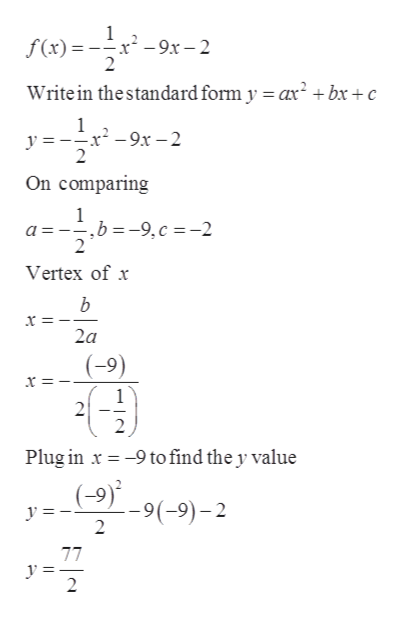# Given the following function (a) find the vertex (b) determine whether there is a maximum or a minimum value and find the value (c) find the range and (d) find the intervals on which the function is increasing and the intervals on which the function is decreasing.f(x)=-1/2x2+9x-2please note that in the above equation i didn't  know how to type a fraction show -1/2x2 means negative one half x squared.

Question
14 views

Given the following function (a) find the vertex (b) determine whether there is a maximum or a minimum value and find the value (c) find the range and (d) find the intervals on which the function is increasing and the intervals on which the function is decreasing.

f(x)=-1/2x2+9x-2

please note that in the above equation i didn't  know how to type a fraction show -1/2x2 means negative one half x squared.

check_circle

Step 1

Note: This question consists of 4 sub parts, we are authorized to answer up to 3 subparts, so we are attempting the first 3 sub parts.

In this question we have a quadratic equation for which we have to finds the vertex, minimum and maximum value and Range.

Step 2

Part A) Vertex

Calculations have shown below,help_outlineImage Transcriptionclose1 f(x)=-9x -2 Write in thestandard form y ar2+ bx +c Er-9x-2 2 On comparing a ,b-9,c = -2 2 Vertex of b 2a x =. 9to find the y value Plug in 99(-9)-2 2 77 y 2 fullscreen
Step 3

Part b)

If we look at the given equation and compare it with the standard equation, then we will find that value of a is negative or less than 0, which means the given f...

### Want to see the full answer?

See Solution

#### Want to see this answer and more?

Solutions are written by subject experts who are available 24/7. Questions are typically answered within 1 hour.*

See Solution
*Response times may vary by subject and question.
Tagged in

### Other